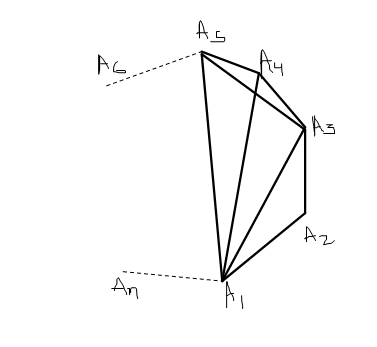Figure not to scale!Suppose $A_{1}$$A_{2}$$A_{3}$$\ldots$$A_{n}$ is an $n$-sided regular polygon such that

$\frac{1}{A_{1}A_{2}}=\frac{1}{A_{1}A_{3}}+\frac{1}{A_{1}A_{4}}.$

Determine the number of sides of the polygon.

×